### Home > CALC > Chapter 3 > Lesson 3.1.2 > Problem3-31

3-31.
1. Evaluate the following limits. Homework Help ✎

1.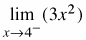2.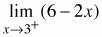3.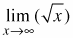4.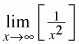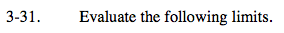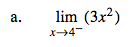Think about the graph of y = 3x². Unlike most one-sided limits, this function approaches the same value from the left as it does from the right. You simply need to evaluate.

3·(4)² = 48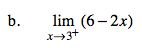Refer to the hint in part (a).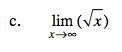$\text{Think about the graph of }y=\sqrt{x}.\text{ What happens as }x\rightarrow \infty ?$

$\text{Is there a horizontal aymptote? If so, the }\lim_{x\rightarrow \infty }\text{ will equal a}$

$\text{constant. Or does the function keep increasing? If so, the}$

$\lim_{x\rightarrow \infty }\text{ will approach }\infty .$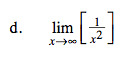Refer to the hint in part (c). Note: you should be able to

$\text{visualize the graph of }y=\frac{1}{x^{2}}.$